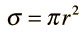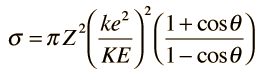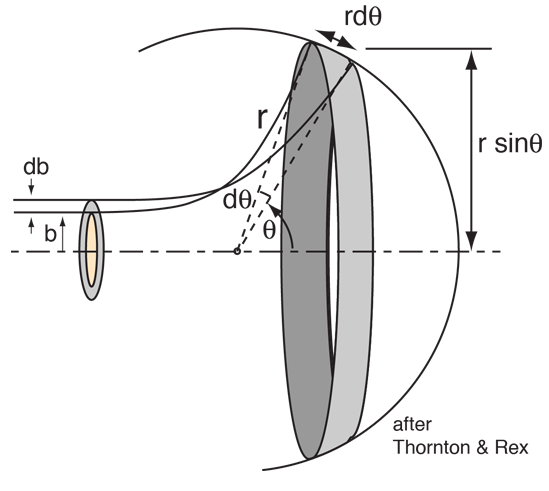# Scattering Cross Section

The concept of cross section, as its name suggests, is that of effective area for collision. The cross section of a spherical target isThe angle of scattering in Rutherford scattering depends upon the impact parameter, with larger deflection occurring for smaller impact parameters. The area of a circle of radius = b = impact parameter is then the cross section for scattering above the angle associated with b, since any particle arriving with r less than b will scatter to a larger angle. Therefore, the cross section for scattering at a greater angle than some chosen angle isNote that this expression is for alpha particles with Zp=2. For projectiles with another charge Zp, then you would multiply this expression by Zp2/4.Calculation of impact parameter and closest approach Determining the cross section and scattered fraction
Index

Rutherford concepts

Reference
Thornton & Rex
HyperPhysics***** Mechanics Go Back